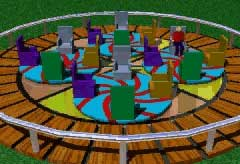Ch 5. Rigid Body General Motion Multimedia Engineering Dynamics Fixed Axis Rotation Plane Motion Velocities Zero Velocity Point Plane Motion Accelerations Multiple Gears Rot. Coord. Velocities Rot. Coord. Acceleration
 Chapter - Particle - 1. General Motion 2. Force & Accel. 3. Energy 4. Momentum - Rigid Body - 5. General Motion 6. Force & Accel. 7. Energy 8. Momentum 9. 3-D Motion 10. Vibrations Appendix Basic Math Units Basic Equations Sections Search eBooks Dynamics Fluids Math Mechanics Statics Thermodynamics Author(s): Kurt Gramoll ©Kurt GramollDYNAMICS - CASE STUDY IntroductionProblem Graphic People riding the spinning-disk ride at Fun Time, Inc. are complaining about high accelerations. An investigation found that the maximum acceleration was occurring at 2.4 seconds. What is known: The main disk rotates at Ω = 1 rad/s (θ = Ωt). The rider disk rotates at ω = 2 rad/s (β = ωt). Both angular velocities are constant. Both disks have no angular acceleration (dω/dt = 0, dΩ/dt = 0). Question What is the acceleration of the rider at 2.4 seconds and is it unsafe? Approach Use the relative acceleration equation for rotating coordinates, aB = aA + dΩ/dt × rB/A + Ω × (Ω × rB/A)              + 2 Ω × vB/A + aB/A

Practice Homework and Test problems now available in the 'Eng Dynamics' mobile app
Includes over 400 problems with complete detailed solutions.
Available now at the Google Play Store and Apple App Store.